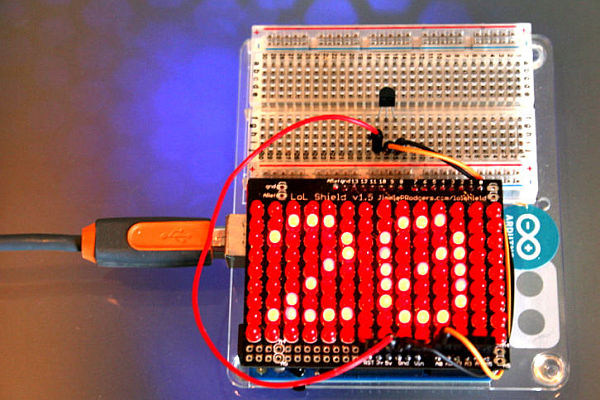# An Arduino Thermometer with Digital Display

This is a project for Arduino to make a Thermometer with Digital Display, aimed at beginners.

Arduino measures the voltage on a TMP36 Temperature sensor, converts the Voltage to Degrees Celcius and Displays the value on an LOL shield display.Objectives:
* Learn how to use the TMP36
* Learn how to perform numeric and string conversions in Arduino-C  (sketch)
* Learn how to display numbers on the LOL shield

## Step 2: Wire it up

Wire the TMP36 as follows:
+5V
GND
SIG => A0

Note: The LOL shield does not come with Headers (as shown in the picture), attached to the topside.

I bought some here: http://www.amazon.co.uk/gp/product/B004RASBVY/ref=oh_details_o06_s00_i00

## Step 3: Install the LOL Shield Libraries

Install the Arduino libraries for the LOL shield if you don’t have them already.You can get the link to them from this page:
http://jimmieprodgers.com/kits/lolshield/

## Step 4: Let’s Start by Reading the Temperature

We’ll start by reading the temperature from the TMP36 and sending the value to the Serial Monitor (Tools -> Serial Monitor on the Arduino IDE).

The TMP36 outputs a voltage on the SIG pin proportional to temperature.

Zero degrees Celcius = 500mV   (0.5)

Each degree above that = 10mV   (1/100)

Here’s a code segment:

float temperature = getVoltage(A0);                        // Get the voltage reading
temperature = (temperature – .5) * 100;                  // Convert to Temperature (degrees C)

## Step 5: Here’s the Full Temperature Reading Code

/*
* Displays Temperature from TMP36 numerically on an LOL shield
* Adapted from (and for more details on this circuit): http://tinyurl.com/c89tvd
*/
//TMP36 Pin Variables
int temperaturePin = 0;
//the analog pin the TMP36’s Vout (sense) pin is connected to
//the resolution is 10 mV / degree centigrade
//(500 mV offset) i.e. 0 degrees = 500mV

/*
* setup() – this function runs once when you turn your Arduino on
* We initialize the serial connection with the computer
*/
void setup()
{
Serial.begin(9600);
//Start the serial connection with the computer
}

void loop()                     // run over and over again
{
float temperature = getVoltage(temperaturePin);
// Get the Voltage reading from the tem

perature sensor
temperature = (temperature – .5) * 100;                 // Convert from 10 mv per degree with 500mV offsetSerial.println(temperature);                     // Print the result
delay(1000);                                             // Wait a second
}

/*
* getVoltage() – returns the voltage on the analog input defined by
* pin
*/
float getVoltage(int pin){
//converting from a 0 to 1023 digital range
// to 0 to 5 volts (each 1 reading equals ~ 5 mV)
}

## Step 6: How to Use the Display

Once you’ve included the library:
#include “Myfont.h”You need to initialise the display in the Setup section:
void setup()
{
LedSign::Init();
}

and Draw the relevant digit in the Loop section:

Myfont::Draw(x_pos, ‘a’);                 // Send a character to the LOL shield

where x_pos is an int holding the x position where you want the number to be display (zero is the left hand edge).

In the final code you will need to convert the temperature reading (a float). To a char so that it can be displayed on the LOL shield using the Draw command.

To do this we use ltoa in C as follows:

temperature = (temperature – .5) * 100;        // Convert to Temperature (a float)

ltoa(long(temperature), buffer, 10);            // buffer is a 12 location char i.e. char buffer;
for (int i=0; i<2; i++) {                                   // Iterate through the buffer for 2 characters
Myfont::Draw(1 + i*6,buffer[i]);                 // Send each character to the LOL shield
}

Notice the x position is:
1 + i*6
this means the first character (i=0) is at x position 1
and their spaced 6 apart (e.g. i=1, x position = 1 + 6 = 7

READ  Web Server with Two Temperature Gauges

Also notice the buffer for ltoa() is 12 long. This is a detail related to the length of long in C and allocation of sufficient memory.

### Major Components in Project

* Arduino UNO
* LOL shield   (http://jimmieprodgers.com/kits/lolshield/)
* TMP36 Temperature Sensing IC
* Breadboard for wiring up e.g. (http://www.oomlout.co.uk/prototyping-bundle-for-arduino-ardp-p-186.html)
* Some Wires

#### This Post / Project can also be found using search terms:

• what does temperature = (temperature – 5) * 100; do in the code?